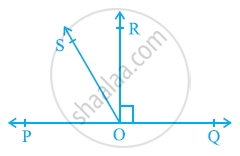# In the given figure, POQ is a line. Ray OR is perpendicular to line PQ. OS is another ray lying between rays OP and OR. Prove that ∠ROS = 1/2(∠QOS - ∠POS). - Mathematics

In the given figure, POQ is a line. Ray OR is perpendicular to line PQ. OS is another ray lying between rays OP and OR. Prove that ∠ROS = 1/2(∠QOS - ∠POS).#### Solution

It is given that OR ⊥ PQ

∴ ∠POR = 90º

⇒ ∠POS + ∠SOR = 90º

∠ROS = 90º − ∠POS … (1)

∠QOR = 90º (As OR ⊥ PQ)

∠QOS − ∠ROS = 90º

∠ROS = ∠QOS − 90º … (2)

On adding equations (1) and (2), we obtain

2 ∠ROS = ∠QOS − ∠POS

∠ROS = 1/2(∠QOS - ∠POS)

Concept: Pairs of Angles
Is there an error in this question or solution?
Chapter 6: Lines and Angles - Exercise 6.1 [Page 97]

#### APPEARS IN

NCERT Class 9 Maths
Chapter 6 Lines and Angles
Exercise 6.1 | Q 5 | Page 97

Share